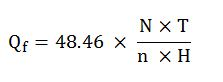## Volume Gain from SlugSlug mud is typically pumped into the drill string in order to push the mud inside the drill pipe down so the drill pipe will be clean and ready for pulling out of hole. Since slug is heavier, it will push the lighter mud out of the well. Figure 1 demonstrates how slug displaces current mud out of hole.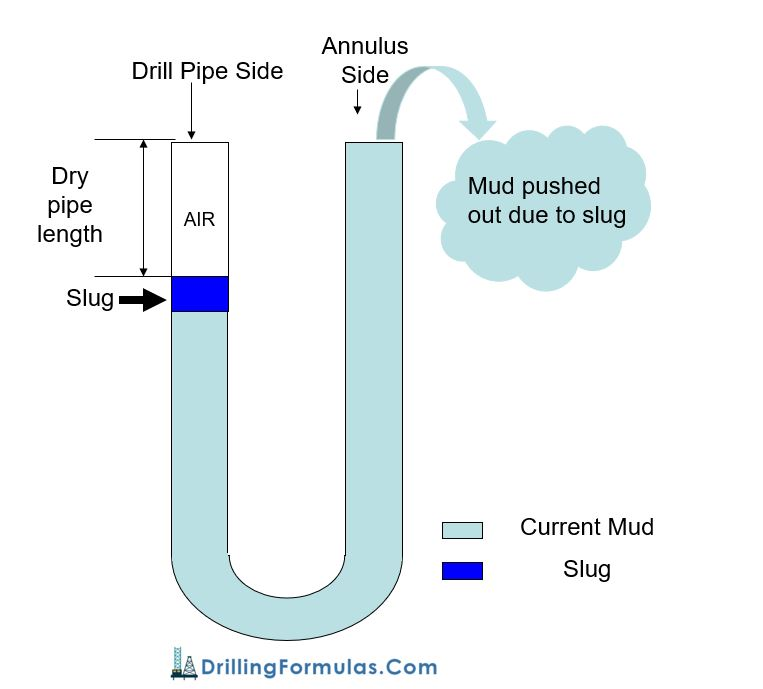Figure 1 – Diagram Shows Volume Gain from Slug

## Determine Bottom Hole Pressure from Wellhead Pressure in a Dry Gas Well

Gas behaves differently from fluid therefore you cannot use a simple hydrostatic formula to determine reservoir pressure. Gas is compressible but fluid is incompressible.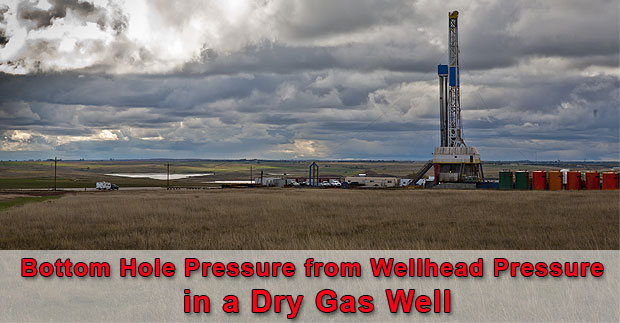The formula to determine the bottom hole pressure of dry gas well is shown below;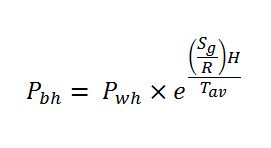Where; Pbh = bottom hole pressure in psia (absolute pressure)

Pwh = wellhead pressure in psia (absolute pressure)

H = true vertical depth of the well

Sg = specific gravity of gas

R = 53.36 ft-lb/lb-R (gas constant for API standard condition air)

Tav = average temperature in Rankin (Rankin = Fahrenheit + 460) Continue reading

## Fracture Gradient Reduction Due to Water Depth

Fracture gradient is one of the critical information which drilling engineers need to know in order to design drilling programs. For the well control stand point, the fracture gradient directly affects on how much influx volume can be successfully contained in the wellbore. If the wellbore pressure is over the fracture pressure, formations would be broken down and this situation will result in loss of drilling fluid into formations. Additionally, it might lead to well control situation because of loss of hydrostatic pressure. Fracture gradient is quite straight forward for land operation because it will not be reduce due to water column. However, the fracture gradient will be reduced in deepwater environment. In this article, we will discuss why water depth can cause the reduction in fracture gradient.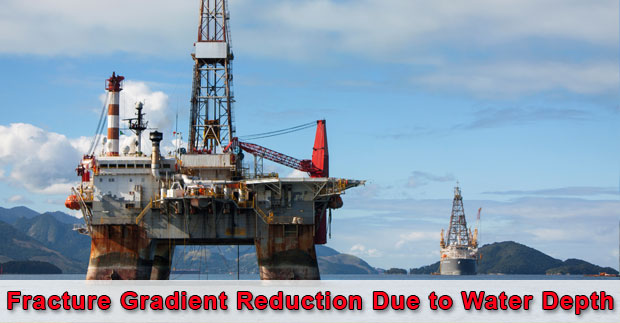Basically, the fracture gradient is related to fluids occupying in pore spaces of rock and weight of rock which are called overburden pressure. Generally, the overburden of a typical sedimentary is about 1.0 psi/ft (19.2 ppg). Rocks will be fractured when the wellbore pressure exceeds the confining stresses acting on it. If we make a general assumption that the overburden pressure causes the minimum confining stress of the rock. Then the formation fracture gradient will not be 1.0 psi/ft if the location is offshore.

Note: this assumption is made in order to help you get more understanding on how and why water depth can decrease the formation fracture gradient.

## Block and Drilling Line Calculation

This article will focus on block and drilling line calculations as block efficiency, drilling power input/output, etc. Additionally, there are some examples which will help you understand how the formulas work.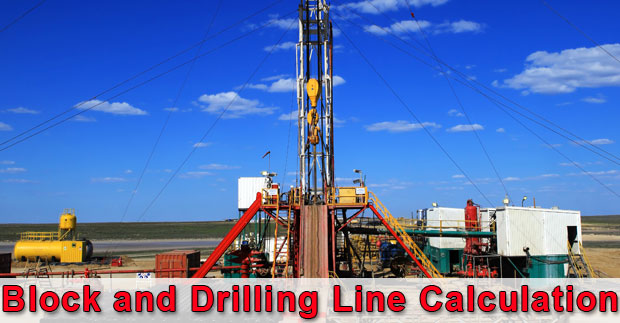Block and drilling line efficiency formula is described below;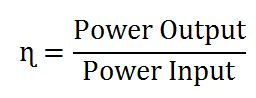Power Output (Po) = Fh x Vtb

Power Input (Pi) = Ff x Vf## Rig Engine Power Consumption and Engine Efficiency

In this article, we will focus on the rig engine power consumption and overall engine efficiency and there are few examples for you to get more understand on how to use the formulas as well.Input Power of Engine

The formula is listed below;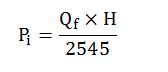Where;

Pi = input power in horse power (hp)

H = fuel heating value in BTU/lb

Qf = fuel consumption rate in lbm/hr

Fuel Consumption

The formula is listed below;